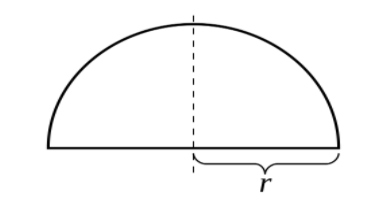QuestionAnswers

# The length of a semicircular tunnel is 2 km and diameter is 7 m. find the expenditure for digging the tunnel at the rate of Rs. 600 per ${m^3}$. Find the expenditure for plastering the inner side of the tunnel at the rate of Rs.50 per ${m^2}$. ($\pi = \dfrac{{{\text{22}}}}{{\text{7}}}$)

Hint: Find the volume of the semicircular tunnel and area of the inner side. Then calculate the expenditure by checking the prices given in the problem. In this problem, we have to calculate two answers.

Complete step by step solution:
To start with, we have the length of the semicircular tunnel, $l$= 2 km = 2000 m.
The diameter of the semicircular tunnel = 7 m.Now, radius of tunnel = $\dfrac{7}{2}$ m = 3.5 m
So, r = 3.5 m, $l$= 2000 m,
Volume of semicircular tunnel, which should be half of the volume of the circular tunnel,
= $\dfrac{1}{2} \times$volume of circular tunnel
= $\dfrac{1}{2} \times$${\pi }{{\text{r}}^{\text{2}}}{\text{l}}$
= $\dfrac{1}{2} \times$$\dfrac{{22}}{7} \times 3.5 \times 3.5 \times 2000$
= $11 \times 0.5 \times 3.5 \times 2000$
= $38500{m^3}$
So, expenditure for digging (semicircular) the tunnel at the rate Rs. 600 per ${m^3}$,
= $38500 \times 600$
= Rs. $2,31,00,000$
Now, for the expenditure for plastering the inner side of the tunnel at the rate Rs. 50 per ${m^2}$,

The inner area of the tunnel = ${\pi rl}$
So, if we calculate, And inner area of the tunnel = ${\pi rl}$= $\dfrac{{22}}{7} \times 3.5 \times 2000$= 22000 ${m^2}$
So total expenditure = 22000$\times$50
= Rs 11,00,000

Note: We always recommend drawing a diagram first to solve a mensuration problem. There are many variations of this particular question. For example, if we talk about the shape then this shape can be half-square or a rectangle or a cylindrical tunnel. these problems are very common in menstruation. Depending upon shape we’ll use the formulas to solve the question.